• 六、分支定界法、 1、整数规划概念、 2、分支定界法求解整数规划步骤、 3、分支定界理论分析、 七、分支过程示例、 八、分支定界法求整数规划示例、 1、分支定界法求整数规划示例、 2、求整数规划的松弛问题及最优解...
文章目录一、整数规划1、整数规划概念2、整数规划分类二、整数规划示例三、整数规划解决的核心问题四、整数规划问题解的特征五、整数规划问题 与 松弛问题 示例六、分支定界法1、整数规划概念2、分支定界法求解整数规划步骤3、分支定界理论分析七、分支过程示例八、分支定界法求整数规划示例1、分支定界法求整数规划示例2、求整数规划的松弛问题及最优解3、第一次分支操作4、第二次分支操作5、第三次分支操作6、整数规划最优解

一、整数规划

1、整数规划概念

线性规划 使用 单纯形法求解 , 线性规划中的 运输规划 使用 表上作业法 求解 ;
之前讨论的都是线性规划问题 , 非线性规划如何求解 , 没有给出具体的方法 ;

整数规划问题 : 要求 一部分 或 全部 决策变量 取值整数 的规划问题 , 称为整数规划 ;
整数规划问题的松弛问题 : 不考虑 整数变量条件 , 剩余的 目标函数 和 约束条件 构成的线性规划问题 称为 整数规划问题的松弛问题 ;
整数线性规划 : 如果上述 整数规划问题的松弛问题 是线性规划  , 则称该整数规划为 整数线性规划 ;

整数规划与之前的线性规划多了一个约束条件 , 变量大于等于 $0$ , 并且都是整数 ;

整数线性规划数学模型一般形式 :
$\begin{array}{lcl} \rm maxZ = \sum_{j = 0}^{n} c_j x_j \\\\ \rm s.t\begin{cases} \rm \sum_{j = 1}^{n} a_{ij} x_j = b_i \ \ \ ( \ i = 1, 2, \cdots , m \ ) \\\\ \rm x_j \geq 0 \ \ \ ( \ j = 1, 2, \cdots , n \ ) 部分 或 全部 为整数 \end{cases}\end{array}$

2、整数规划分类

整数线性规划分为以下几类 : ① 纯整数线性规划 , ② 混合整数线性规划 , ③ 0-1 型整数线性规划 ;

① 纯整数线性规划 : 全部决策变量都 必须取值整数 的 整数线性规划 ;
② 混合整数线性规划 : 决策变量中有一部分 必须 取整数值 , 另一部分 可以不 取值整数值 的 整数线性规划 ;
③ 0-1 型整数线性规划 : 决策变量 只能取值 $0$ 或 $1$ 的整数线性规划 ;

二、整数规划示例

资金总额 $\rm B$ , 有 $n$ 个投资项目 , 项目 $j$ 所需的投资金额 是 $a_j$ , 预期收益是 $c_j$ , $j = 1,2,\cdots,n$ ;
投资还有以下附加条件 :
① 如果投资项目 $1$ , 必须投资项目 $2$ ; 反之如果投资项目 $2$ , 没有限制 ;
② 项目 $3$ 和 项目 $4$ 必须至少选 $1$ 个 ;
③ 项目 $5,6,7$ 只能选择 $2$ 个 ;

决策变量分析 : 选择合适的 决策变量 与 决策变量取值 ;
选取变量 , 使得变量的一组取值 , 能更好对应线性规划问题的解决方案 ;
每个项目有对应的两个选择 , 投资 / 不投资 , 分别使用 $1$ 和 $0$ 表示 ;
令 $x_j$ 表示第 $j$ 个项目的投资选择 , 投资 $1$ , 不投资 $0$ ; ( $j = 1,2, \cdots, n$ )

投资额约束条件 : 所有的投资总额不能超过 $\rm B$ , $\sum_{j = 1}^{n} a_{j} x_j \leq B$ ;
分析条件 ① : 投资项目 $1$ , 必须投资项目 $2$ , 此时 $x_1 = x_2 = 1$ ; 投资项目 $2$ 可以投资项目 $1$ , 可以不投资项目 $1$ , 同时投资的情况上面已经分析过 , 分析后者 $x_1 = 1, x_2 = 0 , 此时 x_1 > x_2$ ; 综合上述两种情况就有 $x_2 \geq x_1$ ;
分析条件 ② : 项目 $3$ 和 项目 $4$ 必须至少选 $1$ 个 , 两者选择一个 , 或者都选择 , 二者相加之和是 $1$ 或 $2$ ; 有约束方程 $x_3 + x_4 \geq 1$ ;
分析条件 ③ : 项目 $5,6,7$ 只能选择 $2$ 个 , 则三者相加等于 $2$ 即可 ; 约束方程 $x_5 + x_6 + x_7 = 2$ ;

投资问题可以表示为以下线性规划 :
$\begin{array}{lcl} \rm maxZ = \sum_{j = 1}^{n} c_j x_j \\\\ \rm s.t\begin{cases} \rm \sum_{j = 1}^{n} a_{j} x_j \leq B \\\\ \rm x_2 \geq x_1 \\\\ \rm x_3 + x_4 \geq 1 \\\\ \rm x_5 + x_6 + x_7 = 2 \\\\ \rm x_j = 0 , 1 \ \ \ ( \ j = 1, 2, \cdots , n \ ) \end{cases}\end{array}$

根据 【运筹学】整数规划 ( 相关概念 | 整数规划 | 整数线性规划 | 整数线性规划分类 ) 博客中的整数线性规划概念 , 上述线性规划是 整数线性规划 ;
上述整数线性规划 的 松弛问题 是一个线性规划 , 可以使用单纯形法对其进行求解 , 求出最优解后 , 可能是小数 , 那么如何得到整数问题的最优解 , 不能进行简单的四舍五入 ;

三、整数规划解决的核心问题

给出 整数规划问题 ,
先求该 整数规划的松弛问题 的解 ,
松弛问题就是不考虑整数约束 , 将整数线性规划当做普通的线性规划 , 使用单纯形法求出其最优解 ;

简单的将其松弛问题最优解上下取整 , 得到的四个值 , 可能 不在可行域中 , 选择的整数解 , 必须在可行域中 ;

根据 整数规划问题的的松弛问题 的最优解 , 如何找其 整数规划问题 的整数最优解 , 是整数规划问题的核心问题 ;

四、整数规划问题解的特征

整数规划问题解的特征 :
① 整数规划问题 与 松弛问题 可行解集合关系 : 整数规划问题 可行解集合 , 是该整数规划问题的 松弛问题 可行解集合 的子集 , 任意两个可行解的 凸组合 , 不一定满足整数约束条件 , 不一定是可行解 ;
② 整数规划问题 与 松弛问题 最优解关系 : 整数规划问题的可行解 一定是 其 松弛问题的可行解 , 松弛问题的可行解不一定是整数规划问题的可行解 , 整数规划问题的最优解 不会优于 松弛问题的最优解 ;

松弛问题 比 整数规划问题 条件少一些 , 整数规划问题比松弛问题变量限制多一条 " 约束变量必须都是整数 " ;

五、整数规划问题 与 松弛问题 示例

假设有如下整数规划问题 :
$\begin{array}{lcl} \rm maxZ = x_1 + x_2 \\\\ \rm s.t\begin{cases} \rm 14 x_1 + 9x_2 \leq 51 \\\\ \rm -6 x_1 + 3x_2 \leq 1 \\\\ \rm x_1, x_2 \geq 0 \ 并且为整数 \end{cases}\end{array}$

上述整数规划问题对应的松弛问题 : 松弛问题 比 整数规划问题 条件少一些 , 整数规划问题比松弛问题变量限制多一条 " 约束变量必须都是整数 " ;
$\begin{array}{lcl} \rm maxZ = x_1 + x_2 \\\\ \rm s.t\begin{cases} \rm 14 x_1 + 9x_2 \leq 51 \\\\ \rm -6 x_1 + 3x_2 \leq 1 \\\\ \rm x_1, x_2 \geq 0 \end{cases}\end{array}$

使用图解法 , 解上述 松弛问题 的最优解为 $\begin{cases} \rm x_1 = \cfrac{3}{2} \\\\ \rm x_2 = \cfrac{10}{3} \end{cases}$
此时目标函数值 $\rm maxZ = x_1 + x_2 = \cfrac{29}{6}$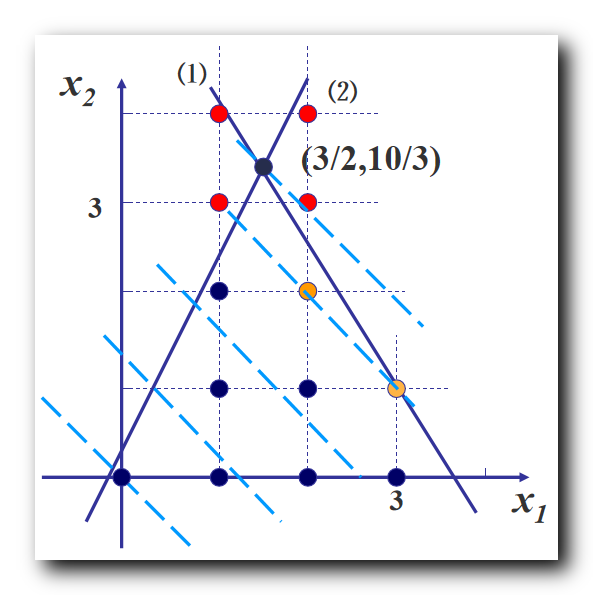简单的将其松弛问题最优解上下取整 , 得到的四个点 , 如上图的四个红色点 ,  都不在可行域中 , 选择的整数解 , 必须在可行域中 ;
根据 整数规划问题的的松弛问题 的最优解 , 如何找其 整数规划问题 的整数最优解 , 是整数规划问题的核心问题 ;

穷举法 ( 有局限性 ) : 直接看上图中可行域内的整数点 , 然后再逐一代入目标函数 , 得到一个 整数规划问题 的最优解  , 但是这种方法无法推广应用 , 如果点的个数比较多 , 如几万个 , 变量的维数多 , 如 $10$ 个约束变量 , 这种方法肯定不适用 ;

整数规划问题的求解方法有 : ① 分支定界法 , ② 割平面法 ;
推荐使用 分支定界法 ;

六、分支定界法

1、整数规划概念

分支定界法相关概念 :
分支 : 将一个问题不断细分为 若干子问题 , 之后逐个讨论子问题 ;
定界 : 分支很多的情况下 , 需要讨论的情况也随之增多 , 这里就需要定界 , 决定在什么时候不在进行分支 ; 满足 ① 得到最优解 , ② 根据现有条件可以排除最优解在该分支中 , 二者其一 , 就可以进行定界 ;
定界的作用是 剪掉没有讨论意义的分支 , 只讨论有意义的分支 ;

2、分支定界法求解整数规划步骤

分支定界法求解整数规划步骤 :
( 1 ) 求 整数规划 的 松弛问题 最优解 :
如果 该 最优解就 是整数 , 那么得到整数规划最优解 ;
如果 该 最优解 不是整数 , 那么转到下一个步骤 分支 与 定界 ;

( 2 ) 分支 与 定界 :
任选一个 非整数解变量 $x_i$ , 在 松弛问题 中加上约束 :
$x_i \leq [x_i]$ 和 $x_i \geq [x_i] + 1$
形成 两个新的 松弛问题 , 就是两个分支 ;
上述分支 , 分的越细致 , 限制条件越多 , 同时 最优解的质量就越差 ;
新的分支松弛问题特征 :

原问题求 最大值 时 , 目标值 是 分支问题 的上界 ;

原问题求 最小值 时 , 目标值 是 分支问题 的下界 ;

分支 $1$ 的最优解是 $x^*$ , 将最优解代入目标函数后得到最优值 $f_1$ ;
分支 $2$ 的最优解是 $y^*$ , 将最优解代入目标函数后得到最优值 $f_2$ ;
如果目标函数求最大值 , 那么就讨论 $f_1, f_2$ 谁更大 ;

检查 分支松弛问题 的 解 及 目标函数值 :
① 得到最优整数解 : 如果该分支的 解 是 整数 , 并且 目标函数值 大于等于 其它分支的目标值 , 则剪去其它分支 , 停止计算 ;
② 没有得到最优整数解 : 如果该分支的 解 是 小数 , 并且 目标函数值 大于 整数解的目标值 , 需要 继续进行分支 , 直到得到最优解 ;

3、分支定界理论分析

假设考虑 分支 $1$ 松弛问题 , 每次都要给问题找到一个界 , 开始先使用观察法找到一个界 , 找到一个整数解 $f$ , 将该解代入目标函数 , 然后在 不断地计算中, 修改该界 ;
假设 分支 $1$ 松弛问题 目标函数 求最大值 ,
如果求解 分支 $1$ 松弛问题 最优解 恰好也是一个整数解 $f_0$ ,  如果 $f_0 > f$ , 此时需要重新定界 , 将 $f_0$ 作为新的界来讨论 ;
根据新的界来看 分支 $2$ 松弛问题是否需要讨论 , 求出 分支 $2$ 的最优解  对应的 目标函数最优值 $f^*$ ;
如果 分支 $2$ 的最优值 小于 分支 $1$ 的最优值 $f^* \leq f^0$ , 此时分支 $2$ 不用再进行分支了 , 再进行分支 , 其最优值会越来越差 ;
定界法的作用是将不符合条件的分支剪去 ;

七、分支过程示例

如上篇博客 【运筹学】整数规划 ( 整数规划问题解的特征 | 整数规划问题 与 松弛问题 示例 ) 中 , 求解如下 整数规划 解 :
$\begin{array}{lcl} \rm maxZ = x_1 + x_2 \\\\ \rm s.t\begin{cases} \rm 14 x_1 + 9x_2 \leq 51 \\\\ \rm -6 x_1 + 3x_2 \leq 1 \\\\ \rm x_1, x_2 \geq 0 \ 并且为整数 \end{cases}\end{array}$

其对应的松弛问题是 : 去掉整数解限制 ;
$\begin{array}{lcl} \rm maxZ = x_1 + x_2 \\\\ \rm s.t\begin{cases} \rm 14 x_1 + 9x_2 \leq 51 \\\\ \rm -6 x_1 + 3x_2 \leq 1 \\\\ \rm x_1, x_2 \geq 0 \end{cases}\end{array}$

分支都是基于松弛问题进行的 , 为松弛问题分支 , 组成两个新的松弛问题 ;

下图是求解结果 ( 图解法 ) :最优解 $x_1 = \cfrac{3}{2}$ , 分别为 $x_1$ 添加 $x_i \leq [x_i]$ 和 $x_i \geq [x_i] + 1$  约束 , 形成两个新的线性规划 ;

分支 $1$ 整数规划 : 添加 $x_i \leq [x_i]$ 约束 , 即 $x_1 \leq 1$ ;
$\begin{array}{lcl} \rm maxZ = x_1 + x_2 \\\\ \rm s.t\begin{cases} \rm 14 x_1 + 9x_2 \leq 51 \\\\ \rm -6 x_1 + 3x_2 \leq 1 \\\\ \rm x_1 \leq 1 \\\\ \rm x_1, x_2 \geq 0 \end{cases}\end{array}$

分支 $2$ 整数规划 : 添加 $x_i \geq [x_i] +1$ 约束 , 即 $x_1 \geq 2$ ;
$\begin{array}{lcl} \rm maxZ = x_1 + x_2 \\\\ \rm s.t\begin{cases} \rm 14 x_1 + 9x_2 \leq 51 \\\\ \rm -6 x_1 + 3x_2 \leq 1 \\\\ \rm x_1 \geq 2 \\\\ \rm x_1, x_2 \geq 0 \end{cases}\end{array}$

这样就将一个线性规划 , 分解成了两个问题分支 , 分别找两个分支问题的所有整数解 ;
整数规划的整数解 , 肯定在上述两个分支之一中 , 中间将一部分可行域排除在外了 , 就是下图中两个红色箭头之间的可行域部分 , 被排除掉的部分肯定没有整数解 , 都是小数 ;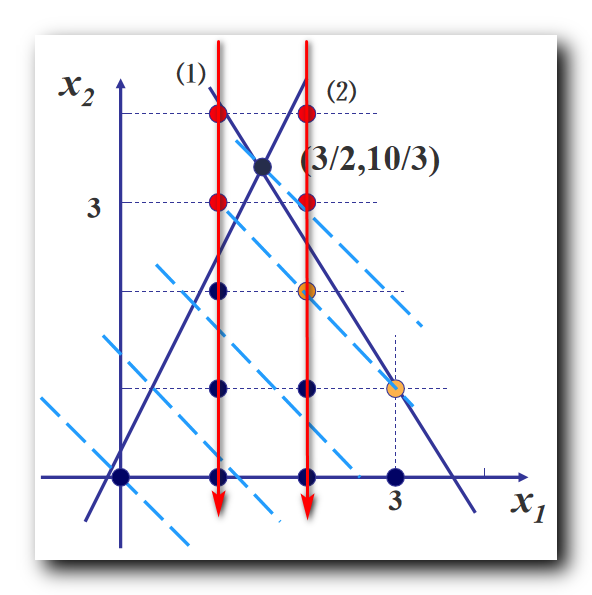八、分支定界法求整数规划示例

1、分支定界法求整数规划示例

使用 分支定界法 求如下整数规划 :
$\begin{array}{lcl} \rm min W = -x_1 -5 x_2 \\\\ \rm s.t\begin{cases} \rm x_1 - x_2 \geq -2 \\\\ \rm 5x_1 + 6x_2 \leq 30 \\\\ \rm x_1 \leq 4 \\\\ \rm x_1, x_2 \geq 0 \ 并且为整数 \end{cases}\end{array}$

2、求整数规划的松弛问题及最优解

求上述整数规划 ( $\rm IP$ ) 的松弛问题 ( $\rm LP$ ) : 去掉整数限制即可得到一个一般的线性规划 ;
$\begin{array}{lcl} \rm min W = -x_1 -5 x_2 \\\\ \rm s.t\begin{cases} \rm x_1 - x_2 \geq -2 \\\\ \rm 5x_1 + 6x_2 \leq 30 \\\\ \rm x_1 \leq 4 \\\\ \rm x_1, x_2 \geq 0 \end{cases}\end{array}$
可以使用单纯形法求上述线性规划的最优解 , 本次使用图示法 , 求的最优化 ;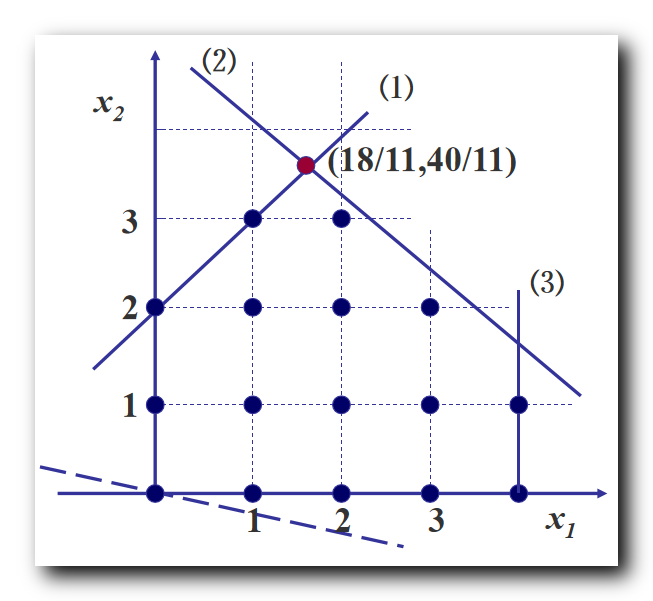使用图示法解得上述 松弛问题 最优解 $\begin{cases} \rm x_1 = \cfrac{18}{11} \approx 1.64 \\\\ \rm x_2 = \cfrac{40}{11} \approx 3.64 \end{cases}$ , 将上述最优解代入约束方程 ,
得到整数规划最小值 $\rm min W = -x_1 -5 x_2 = - \cfrac{218}{11} \approx -19.8$

如果上述 松弛问题 最优解 是整数 , 则该整数线性规划的最优解就是 松弛问题 的最优解 ;
上述 松弛问题 $\rm LP$ 最优解不是整数 , 这里需要进行 分支 操作 , 分成两个 分支松弛问题 $\rm LP1$ 和 $\rm LP2$ ;

3、第一次分支操作

分支操作 : 任选一个 非整数解变量 $x_i$ , 在 松弛问题 中加上约束 , $x_i \leq [x_i]$ 和 $x_i \geq [x_i] + 1$  , 形成 两个新的 松弛问题 , 就是两个分支 ;

选择 非整数取值的变量 $x_1 = \cfrac{18}{11} \approx 1.64$ , 作为分支变量 ,
$x_i \leq [x_i]$ 对应的 分支新增约束条件是 $x_1 \leq 1$ ;
$x_i \geq [x_i] + 1$ 对应的 分支新增约束条件是 $x_1 \geq 2$ ;

在 $\rm LP1$ 分支松弛问题中加入 $x_1 \leq 1$ 条件后为 :
$\begin{array}{lcl} \rm min W = -x_1 -5 x_2 \\\\ \rm s.t\begin{cases} \rm x_1 - x_2 \geq -2 \\\\ \rm 5x_1 + 6x_2 \leq 30 \\\\ \rm x_1 \leq 4 \\\\ \rm x_1 \leq 1 \\\\ \rm x_1, x_2 \geq 0 \end{cases}\end{array}$
求上述 $\rm LP1$ 分支的最优解  $\begin{cases} \rm x_1 = 1 \\\\ \rm x_2 = 3 \end{cases}$ , 将上述最优解代入约束方程 ,
得到整数规划最小值 $\rm min W = -x_1 -5 x_2 = - 16$
$\rm LP1$ 分支的界就是 $-16$ ;

在 $\rm LP2$ 分支松弛问题中加入 $x_1 \geq 2$ 条件后为 :
$\begin{array}{lcl} \rm min W = -x_1 -5 x_2 \\\\ \rm s.t\begin{cases} \rm x_1 - x_2 \geq -2 \\\\ \rm 5x_1 + 6x_2 \leq 30 \\\\ \rm x_1 \leq 4 \\\\ \rm x_1 \geq 2 \\\\ \rm x_1, x_2 \geq 0 \end{cases}\end{array}$
求上述 $\rm LP2$ 分支的最优解  $\begin{cases} \rm x_1 = 2 \\\\ \rm x_2 = \cfrac{10}{3} \end{cases}$ , 将上述最优解代入约束方程 ,
得到整数规划最小值 $\rm min W = -x_1 -5 x_2 = - \cfrac{56}{3} \approx -18.7$
$\rm LP2$ 分支的目标值是 $-18.7$ ;

$\rm LP1$ 分支的最优解时整数 , 界 是 $-16$ ,
$\rm LP2$ 分支目标值还不是整数 , 因此需要继续分支 ;

判定某个分支 松弛问题 是否继续向下分支的依据 :
① 根据整最优解是否是整数判定 : 首先看 分支 松弛问题 最优解 是否是整数 , 如果是那就停下来 , 如果不是继续向下分支 ;
② 根据界的优劣判定 ( 定界思想 ) : 是否继续向下分支 , 还需要看 界 的值 , 通过该 界 的值 , 讨论是否继续向下分支 ;

分支条件 : 如果 本分支的界 比 另外一个分支的界  要好 , 则继续分支下去 ;
不分支条件 : 如果本分支的界比另外一个分支的界差 , 那么本分支就不再向下分支了 ;

4、第二次分支操作

$\rm LP2$ 继续向下分支 , $x_1$ 变量已经是整数变量了 ,
这里 选择 非整数取值的变量 $x_2 = \cfrac{10}{3} \approx 3.33$ , 作为分支变量 ,
$x_i \leq [x_i]$ 对应的 分支新增约束条件是 $x_2 \leq 3$ ;
$x_i \geq [x_i] + 1$ 对应的 分支新增约束条件是 $x_2 \geq 4$ ;

这里得到了 $\rm LP2$ 分支下的两个 分支松弛问题 $\rm LP21$ 和 $\rm LP22$ ;
在 $\rm LP21$ 分支松弛问题中加入 $x_2 \leq 3$ 条件后为 :
$\begin{array}{lcl} \rm min W = -x_1 -5 x_2 \\\\ \rm s.t\begin{cases} \rm x_1 - x_2 \geq -2 \\\\ \rm 5x_1 + 6x_2 \leq 30 \\\\ \rm x_1 \leq 4 \\\\ \rm x_1 \geq 2 \\\\ \rm x_2 \leq 3 \\\\ \rm x_1, x_2 \geq 0 \end{cases}\end{array}$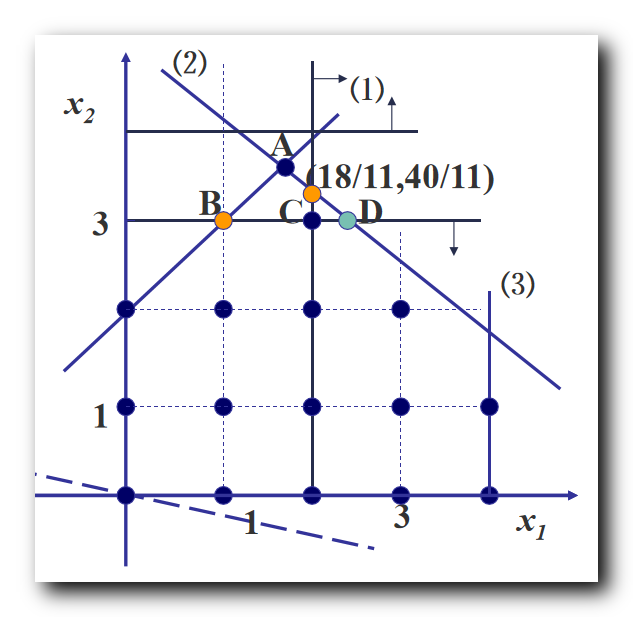求上述 $\rm LP21$ 分支的最优解  $\begin{cases} \rm x_1 = \cfrac{12}{5} = 2.4 \\\\ \rm x_2 = 3 \end{cases}$ , 将上述最优解代入约束方程 ,
得到整数规划最小值 $\rm min W = -x_1 -5 x_2 = - 17.4$
$\rm LP21$ 分支的最优解不是整数 , 而且比 $\rm LP1$ 分支的界 $-16$ 要好 , 可需要继续分支 ;

在 $\rm LP22$ 分支松弛问题中加入 $x_2 \geq 4$ 条件后为 :
$\begin{array}{lcl} \rm min W = -x_1 -5 x_2 \\\\ \rm s.t\begin{cases} \rm x_1 - x_2 \geq -2 \\\\ \rm 5x_1 + 6x_2 \leq 30 \\\\ \rm x_1 \leq 4 \\\\ \rm x_1 \geq 2 \\\\ \rm x_2 \geq 4 \\\\ \rm x_1, x_2 \geq 0 \end{cases}\end{array}$
上述 $\rm LP22$ 分支 没有可行解 , 直接停止 ;

5、第三次分支操作

$\rm LP21$ 继续向下分支 ,
这里 选择 非整数取值的变量 $x_1 = \cfrac{12}{5} = 2.4$ , 作为分支变量 ,
$x_i \leq [x_i]$ 对应的 分支新增约束条件是 $x_1 \leq 2$ ;
$x_i \geq [x_i] + 1$ 对应的 分支新增约束条件是 $x_1 \geq 3$ ;
这里得到了 $\rm LP21$ 分支下的两个 分支松弛问题 $\rm LP211$ 和 $\rm LP212$ ;

在 $\rm LP211$ 分支松弛问题中加入 $x_1 \leq 2$ 条件后为 :
$\begin{array}{lcl} \rm min W = -x_1 -5 x_2 \\\\ \rm s.t\begin{cases} \rm x_1 - x_2 \geq -2 \\\\ \rm 5x_1 + 6x_2 \leq 30 \\\\ \rm x_1 \leq 4 \\\\ \rm x_1 \geq 2 \\\\ \rm x_1 \leq 2 \\\\ \rm x_2 \leq 3 \\\\ \rm x_1, x_2 \geq 0 \end{cases}\end{array}$
求上述 $\rm LP211$ 分支的最优解  $\begin{cases} \rm x_1 = 2 \\\\ \rm x_2 = 3 \end{cases}$ , 将上述最优解代入约束方程 ,
得到整数规划最小值 $\rm min W = -x_1 -5 x_2 = - 17$
$\rm LP21$ 分支的最优解是整数 , 而且比 $\rm LP1$ 分支的界 $-16$ 要好 , 是当前最好的 界 ;
因此这里将 界 更新为 $-17$ ;

在 $\rm LP212$ 分支松弛问题中加入 $x_1 \geq 3$ 条件后为 :
$\begin{array}{lcl} \rm min W = -x_1 -5 x_2 \\\\ \rm s.t\begin{cases} \rm x_1 - x_2 \geq -2 \\\\ \rm 5x_1 + 6x_2 \leq 30 \\\\ \rm x_1 \leq 4 \\\\ \rm x_1 \geq 2 \\\\ \rm x_1 \geq 3 \\\\ \rm x_2 \leq 3 \\\\ \rm x_1, x_2 \geq 0 \end{cases}\end{array}$
求上述 $\rm LP212$ 分支的最优解  $\begin{cases} \rm x_1 =3 \\\\ \rm x_2 = 2.5 \end{cases}$ , 将上述最优解代入约束方程 ,
得到整数规划最小值 $\rm min W = -x_1 -5 x_2 = - 15.5$
定界 : $\rm LP212$ 分支的最优解不是整数 , 其目标值 $- 15.5$ 要比当前的界 $-17$ 要差 , 因此该分支直接裁减掉 ;

6、整数规划最优解

该整数规划 $\rm IP$ 的最优解  $\begin{cases} \rm x_1 = 2 \\\\ \rm x_2 = 3 \end{cases}$ , 将上述最优解代入约束方程 ,
得到整数规划最小值 $\rm min W = -x_1 -5 x_2 = - 17$

分支记录如下 :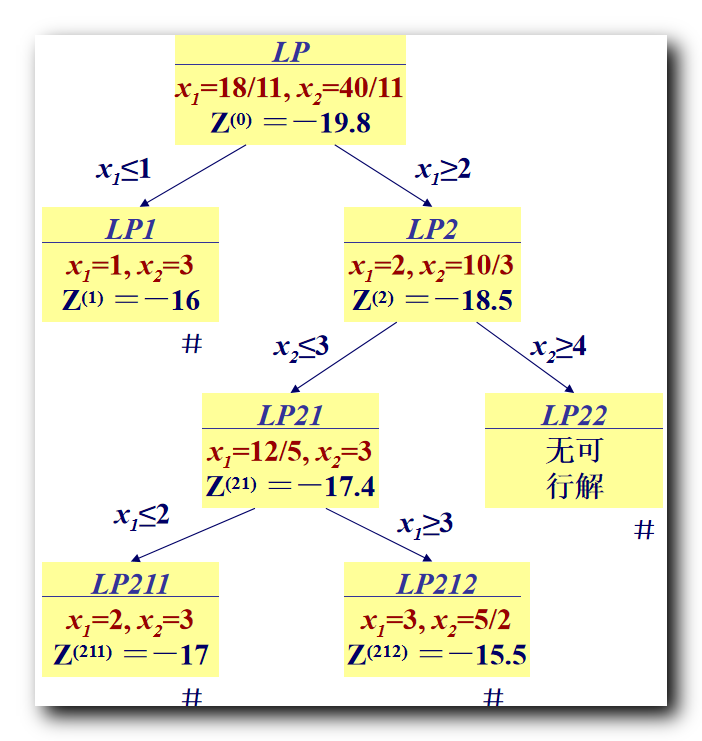展开全文整数规划
• 分支定界法（branch and bound）是一种求解离散数据组合的最优化问题。该算法执行的效率取决于你所找的问题解空间的上下界，如果找到一个很紧凑的上下界进行剪枝操作，该算法的执行效率会非常高，因此它是最有可能在...
分支定界法（branch and bound）是一种求解离散数据组合的最优化问题。该算法执行的效率取决于你所找的问题解空间的上下界，如果找到一个很紧凑的上下界进行剪枝操作，该算法的执行效率会非常高，因此它是最有可能在多项式时间内求解NP问题的算法。
使用分支定界算法的一般步骤为：
构造一棵搜索树，该搜索树指的是所有解空间，因此通过遍历该搜索树可以遍历到所有的解；
构造问题解的上下界，上界一般为之前求出的最优解，下界为无约束条件下当前搜索路径的最优解，上下界的主要作用是对搜索树进行剪枝；
通过回溯法遍历搜索树，并且不断更新上下界，如果当前解的下界已经超过上界，则进行剪枝；
遍历结束时，所求的解为最优解。
接下来通过一个实例来讲解分支定界算法：
某公司于乙城市的销售点急需一批成品，该公司成品生产基地在甲城市。 甲城市与乙城市之间共有 n 座城市，互相以公路连通。甲城市、乙城市以及其它各城市之间的公路连通情况及每段公路的长度由矩阵M1 给出。 每段公路均由地方政府收取不同额度的养路费等费用， 具体数额由矩阵 M2 给出。 请给出在需付养路费总额不超过 1500 的情况下，该公司货车运送其产品从甲城市到乙城市的最短运送路线。(题目来源：北航研究生算法课)
首先构造一棵搜索树，该搜索树并不需要显示的构建，而是在搜索过程中所遵循的一种搜索规则。对于上述问题，以甲城市为根节点构建二叉树，其它节点由剩余城市表示，树的左子树表示当前路径包含该父节点，树的右子树表示当前路径不包含该父节点。如图所示该搜索路径所表示的实际路径为1-3-4，即路径中不包含城市2。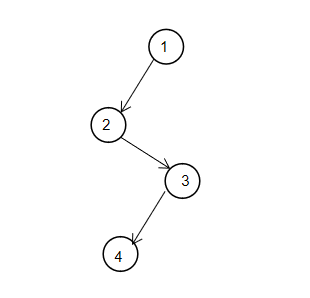然后分析该问题解的上下界：搜索路径的上界为当前已经求出的满足条件的最短路径长度。搜索路径的下界为当前路径长度与无约束条件下路径终点到城市乙的最短路径长度之和。若上界大于下界，则可以继续搜索；若上界小于下界，则表示无更优解，此时可进行剪枝操作。其中无约束条件下的任意点到城市乙的最短路径长度可以使用Dijkstra或Floyd算法预先求出。
最后通过深度优先遍历该搜索树，以及通过上下界进行剪枝对该问题进行求解。如下是该问题的Python实现，程序运行的结果为：最终的路径为0-2-7-10-14-20-22-25-31-36-38-44-46-49，该路径长度为464，所需要的总的养路费为1448。程序运行的总时间大约为0.01s。如果有兴趣可以将该解的上下界修改为更宽的上下界，可以很明显的看出程序的运行时间增加。
注：该问题的数据和代码可以在我的GitHub中获取。

转载于:https://www.cnblogs.com/alants/p/10443895.html
展开全文• 一、分支定界法相关概念、 二、分支定界法求解整数规划步骤、 三、分支定界理论分析、 四、分支过程示例
文章目录一、分支定界法相关概念二、分支定界法求解整数规划步骤三、分支定界理论分析四、分支过程示例

一、分支定界法相关概念

分支定界法相关概念 :
分支 : 将一个问题不断细分为 若干子问题 , 之后逐个讨论子问题 ;
定界 : 分支很多的情况下 , 需要讨论的情况也随之增多 , 这里就需要定界 , 决定在什么时候不在进行分支 ; 满足 ① 得到最优解 , ② 根据现有条件可以排除最优解在该分支中 , 二者其一 , 就可以进行定界 ;
定界的作用是 剪掉没有讨论意义的分支 , 只讨论有意义的分支 ;

二、分支定界法求解整数规划步骤

分支定界法求解整数规划步骤 :
( 1 ) 求 整数规划 的 松弛问题 最优解 :
如果 该 最优解就 是整数 , 那么得到整数规划最优解 ;
如果 该 最优解 不是整数 , 那么转到下一个步骤 分支 与 定界 ;

( 2 ) 分支 与 定界 :
任选一个 非整数解变量 $x_i$ , 在 松弛问题 中加上约束 :
$x_i \leq [x_i]$ 和 $x_i \geq [x_i] + 1$
形成 两个新的 松弛问题 , 就是两个分支 ;
上述分支 , 分的越细致 , 限制条件越多 , 同时 最优解的质量就越差 ;
新的分支松弛问题特征 :

原问题求 最大值 时 , 目标值 是 分支问题 的上界 ;

原问题求 最小值 时 , 目标值 是 分支问题 的下界 ;

分支 $1$ 的最优解是 $x^*$ , 将最优解代入目标函数后得到最优值 $f_1$ ;
分支 $2$ 的最优解是 $y^*$ , 将最优解代入目标函数后得到最优值 $f_2$ ;
如果目标函数求最大值 , 那么就讨论 $f_1, f_2$ 谁更大 ;

检查 分支松弛问题 的 解 及 目标函数值 :
① 得到最优整数解 : 如果该分支的 解 是 整数 , 并且 目标函数值 大于等于 其它分支的目标值 , 则剪去其它分支 , 停止计算 ;
② 没有得到最优整数解 : 如果该分支的 解 是 小数 , 并且 目标函数值 大于 整数解的目标值 , 需要 继续进行分支 , 直到得到最优解 ;

三、分支定界理论分析

假设考虑 分支 $1$ 松弛问题 , 每次都要给问题找到一个界 , 开始先使用观察法找到一个界 , 找到一个整数解 $f$ , 将该解代入目标函数 , 然后在 不断地计算中, 修改该界 ;
假设 分支 $1$ 松弛问题 目标函数 求最大值 ,
如果求解 分支 $1$ 松弛问题 最优解 恰好也是一个整数解 $f_0$ ,  如果 $f_0 > f$ , 此时需要重新定界 , 将 $f_0$ 作为新的界来讨论 ;
根据新的界来看 分支 $2$ 松弛问题是否需要讨论 , 求出 分支 $2$ 的最优解  对应的 目标函数最优值 $f^*$ ;
如果 分支 $2$ 的最优值 小于 分支 $1$ 的最优值 $f^* \leq f^0$ , 此时分支 $2$ 不用再进行分支了 , 再进行分支 , 其最优值会越来越差 ;
定界法的作用是将不符合条件的分支剪去 ;

四、分支过程示例

如上篇博客 【运筹学】整数规划 ( 整数规划问题解的特征 | 整数规划问题 与 松弛问题 示例 ) 中 , 求解如下 整数规划 解 :
$\begin{array}{lcl} \rm maxZ = x_1 + x_2 \\\\ \rm s.t\begin{cases} \rm 14 x_1 + 9x_2 \leq 51 \\\\ \rm -6 x_1 + 3x_2 \leq 1 \\\\ \rm x_1, x_2 \geq 0 \ 并且为整数 \end{cases}\end{array}$

其对应的松弛问题是 : 去掉整数解限制 ;
$\begin{array}{lcl} \rm maxZ = x_1 + x_2 \\\\ \rm s.t\begin{cases} \rm 14 x_1 + 9x_2 \leq 51 \\\\ \rm -6 x_1 + 3x_2 \leq 1 \\\\ \rm x_1, x_2 \geq 0 \end{cases}\end{array}$

分支都是基于松弛问题进行的 , 为松弛问题分支 , 组成两个新的松弛问题 ;

下图是求解结果 ( 图解法 ) :最优解 $x_1 = \cfrac{3}{2}$ , 分别为 $x_1$ 添加 $x_i \leq [x_i]$ 和 $x_i \geq [x_i] + 1$  约束 , 形成两个新的线性规划 ;

分支 $1$ 整数规划 : 添加 $x_i \leq [x_i]$ 约束 , 即 $x_1 \leq 1$ ;
$\begin{array}{lcl} \rm maxZ = x_1 + x_2 \\\\ \rm s.t\begin{cases} \rm 14 x_1 + 9x_2 \leq 51 \\\\ \rm -6 x_1 + 3x_2 \leq 1 \\\\ \rm x_1 \leq 1 \\\\ \rm x_1, x_2 \geq 0 \end{cases}\end{array}$

分支 $2$ 整数规划 : 添加 $x_i \geq [x_i] +1$ 约束 , 即 $x_1 \geq 2$ ;
$\begin{array}{lcl} \rm maxZ = x_1 + x_2 \\\\ \rm s.t\begin{cases} \rm 14 x_1 + 9x_2 \leq 51 \\\\ \rm -6 x_1 + 3x_2 \leq 1 \\\\ \rm x_1 \geq 2 \\\\ \rm x_1, x_2 \geq 0 \end{cases}\end{array}$

这样就将一个线性规划 , 分解成了两个问题分支 , 分别找两个分支问题的所有整数解 ;
整数规划的整数解 , 肯定在上述两个分支之一中 , 中间将一部分可行域排除在外了 , 就是下图中两个红色箭头之间的可行域部分 , 被排除掉的部分肯定没有整数解 , 都是小数 ;展开全文整数规划问题
• 一、分支定界法求整数规划示例、 二、求整数规划的松弛问题及最优解、 三、第一次分支操作、 四、第二次分支操作、 五、第三次分支操作、 六、整数规划最优解
文章目录一、分支定界法求整数规划示例二、求整数规划的松弛问题及最优解三、第一次分支操作四、第二次分支操作五、第三次分支操作六、整数规划最优解

一、分支定界法求整数规划示例

使用 分支定界法 求如下整数规划 :
$\begin{array}{lcl} \rm min W = -x_1 -5 x_2 \\\\ \rm s.t\begin{cases} \rm x_1 - x_2 \geq -2 \\\\ \rm 5x_1 + 6x_2 \leq 30 \\\\ \rm x_1 \leq 4 \\\\ \rm x_1, x_2 \geq 0 \ 并且为整数 \end{cases}\end{array}$

二、求整数规划的松弛问题及最优解

求上述整数规划 ( $\rm IP$ ) 的松弛问题 ( $\rm LP$ ) : 去掉整数限制即可得到一个一般的线性规划 ;
$\begin{array}{lcl} \rm min W = -x_1 -5 x_2 \\\\ \rm s.t\begin{cases} \rm x_1 - x_2 \geq -2 \\\\ \rm 5x_1 + 6x_2 \leq 30 \\\\ \rm x_1 \leq 4 \\\\ \rm x_1, x_2 \geq 0 \end{cases}\end{array}$
可以使用单纯形法求上述线性规划的最优解 , 本次使用图示法 , 求的最优化 ;使用图示法解得上述 松弛问题 最优解 $\begin{cases} \rm x_1 = \cfrac{18}{11} \approx 1.64 \\\\ \rm x_2 = \cfrac{40}{11} \approx 3.64 \end{cases}$ , 将上述最优解代入约束方程 ,
得到整数规划最小值 $\rm min W = -x_1 -5 x_2 = - \cfrac{218}{11} \approx -19.8$

如果上述 松弛问题 最优解 是整数 , 则该整数线性规划的最优解就是 松弛问题 的最优解 ;
上述 松弛问题 $\rm LP$ 最优解不是整数 , 这里需要进行 分支 操作 , 分成两个 分支松弛问题 $\rm LP1$ 和 $\rm LP2$ ;

三、第一次分支操作

分支操作 : 任选一个 非整数解变量 $x_i$ , 在 松弛问题 中加上约束 , $x_i \leq [x_i]$ 和 $x_i \geq [x_i] + 1$  , 形成 两个新的 松弛问题 , 就是两个分支 ;

选择 非整数取值的变量 $x_1 = \cfrac{18}{11} \approx 1.64$ , 作为分支变量 ,
$x_i \leq [x_i]$ 对应的 分支新增约束条件是 $x_1 \leq 1$ ;
$x_i \geq [x_i] + 1$ 对应的 分支新增约束条件是 $x_1 \geq 2$ ;

在 $\rm LP1$ 分支松弛问题中加入 $x_1 \leq 1$ 条件后为 :
$\begin{array}{lcl} \rm min W = -x_1 -5 x_2 \\\\ \rm s.t\begin{cases} \rm x_1 - x_2 \geq -2 \\\\ \rm 5x_1 + 6x_2 \leq 30 \\\\ \rm x_1 \leq 4 \\\\ \rm x_1 \leq 1 \\\\ \rm x_1, x_2 \geq 0 \end{cases}\end{array}$
求上述 $\rm LP1$ 分支的最优解  $\begin{cases} \rm x_1 = 1 \\\\ \rm x_2 = 3 \end{cases}$ , 将上述最优解代入约束方程 ,
得到整数规划最小值 $\rm min W = -x_1 -5 x_2 = - 16$
$\rm LP1$ 分支的界就是 $-16$ ;

在 $\rm LP2$ 分支松弛问题中加入 $x_1 \geq 2$ 条件后为 :
$\begin{array}{lcl} \rm min W = -x_1 -5 x_2 \\\\ \rm s.t\begin{cases} \rm x_1 - x_2 \geq -2 \\\\ \rm 5x_1 + 6x_2 \leq 30 \\\\ \rm x_1 \leq 4 \\\\ \rm x_1 \geq 2 \\\\ \rm x_1, x_2 \geq 0 \end{cases}\end{array}$
求上述 $\rm LP2$ 分支的最优解  $\begin{cases} \rm x_1 = 2 \\\\ \rm x_2 = \cfrac{10}{3} \end{cases}$ , 将上述最优解代入约束方程 ,
得到整数规划最小值 $\rm min W = -x_1 -5 x_2 = - \cfrac{56}{3} \approx -18.7$
$\rm LP2$ 分支的目标值是 $-18.7$ ;

$\rm LP1$ 分支的最优解时整数 , 界 是 $-16$ ,
$\rm LP2$ 分支目标值还不是整数 , 因此需要继续分支 ;

判定某个分支 松弛问题 是否继续向下分支的依据 :
① 根据整最优解是否是整数判定 : 首先看 分支 松弛问题 最优解 是否是整数 , 如果是那就停下来 , 如果不是继续向下分支 ;
② 根据界的优劣判定 ( 定界思想 ) : 是否继续向下分支 , 还需要看 界 的值 , 通过该 界 的值 , 讨论是否继续向下分支 ;

分支条件 : 如果 本分支的界 比 另外一个分支的界  要好 , 则继续分支下去 ;
不分支条件 : 如果本分支的界比另外一个分支的界差 , 那么本分支就不再向下分支了 ;

四、第二次分支操作

$\rm LP2$ 继续向下分支 , $x_1$ 变量已经是整数变量了 ,
这里 选择 非整数取值的变量 $x_2 = \cfrac{10}{3} \approx 3.33$ , 作为分支变量 ,
$x_i \leq [x_i]$ 对应的 分支新增约束条件是 $x_2 \leq 3$ ;
$x_i \geq [x_i] + 1$ 对应的 分支新增约束条件是 $x_2 \geq 4$ ;

这里得到了 $\rm LP2$ 分支下的两个 分支松弛问题 $\rm LP21$ 和 $\rm LP22$ ;
在 $\rm LP21$ 分支松弛问题中加入 $x_2 \leq 3$ 条件后为 :
$\begin{array}{lcl} \rm min W = -x_1 -5 x_2 \\\\ \rm s.t\begin{cases} \rm x_1 - x_2 \geq -2 \\\\ \rm 5x_1 + 6x_2 \leq 30 \\\\ \rm x_1 \leq 4 \\\\ \rm x_1 \geq 2 \\\\ \rm x_2 \leq 3 \\\\ \rm x_1, x_2 \geq 0 \end{cases}\end{array}$求上述 $\rm LP21$ 分支的最优解  $\begin{cases} \rm x_1 = \cfrac{12}{5} = 2.4 \\\\ \rm x_2 = 3 \end{cases}$ , 将上述最优解代入约束方程 ,
得到整数规划最小值 $\rm min W = -x_1 -5 x_2 = - 17.4$
$\rm LP21$ 分支的最优解不是整数 , 而且比 $\rm LP1$ 分支的界 $-16$ 要好 , 可需要继续分支 ;

在 $\rm LP22$ 分支松弛问题中加入 $x_2 \geq 4$ 条件后为 :
$\begin{array}{lcl} \rm min W = -x_1 -5 x_2 \\\\ \rm s.t\begin{cases} \rm x_1 - x_2 \geq -2 \\\\ \rm 5x_1 + 6x_2 \leq 30 \\\\ \rm x_1 \leq 4 \\\\ \rm x_1 \geq 2 \\\\ \rm x_2 \geq 4 \\\\ \rm x_1, x_2 \geq 0 \end{cases}\end{array}$
上述 $\rm LP22$ 分支 没有可行解 , 直接停止 ;

五、第三次分支操作

$\rm LP21$ 继续向下分支 ,
这里 选择 非整数取值的变量 $x_1 = \cfrac{12}{5} = 2.4$ , 作为分支变量 ,
$x_i \leq [x_i]$ 对应的 分支新增约束条件是 $x_1 \leq 2$ ;
$x_i \geq [x_i] + 1$ 对应的 分支新增约束条件是 $x_1 \geq 3$ ;
这里得到了 $\rm LP21$ 分支下的两个 分支松弛问题 $\rm LP211$ 和 $\rm LP212$ ;

在 $\rm LP211$ 分支松弛问题中加入 $x_1 \leq 2$ 条件后为 :
$\begin{array}{lcl} \rm min W = -x_1 -5 x_2 \\\\ \rm s.t\begin{cases} \rm x_1 - x_2 \geq -2 \\\\ \rm 5x_1 + 6x_2 \leq 30 \\\\ \rm x_1 \leq 4 \\\\ \rm x_1 \geq 2 \\\\ \rm x_1 \leq 2 \\\\ \rm x_2 \leq 3 \\\\ \rm x_1, x_2 \geq 0 \end{cases}\end{array}$
求上述 $\rm LP211$ 分支的最优解  $\begin{cases} \rm x_1 = 2 \\\\ \rm x_2 = 3 \end{cases}$ , 将上述最优解代入约束方程 ,
得到整数规划最小值 $\rm min W = -x_1 -5 x_2 = - 17$
$\rm LP21$ 分支的最优解是整数 , 而且比 $\rm LP1$ 分支的界 $-16$ 要好 , 是当前最好的 界 ;
因此这里将 界 更新为 $-17$ ;

在 $\rm LP212$ 分支松弛问题中加入 $x_1 \geq 3$ 条件后为 :
$\begin{array}{lcl} \rm min W = -x_1 -5 x_2 \\\\ \rm s.t\begin{cases} \rm x_1 - x_2 \geq -2 \\\\ \rm 5x_1 + 6x_2 \leq 30 \\\\ \rm x_1 \leq 4 \\\\ \rm x_1 \geq 2 \\\\ \rm x_1 \geq 3 \\\\ \rm x_2 \leq 3 \\\\ \rm x_1, x_2 \geq 0 \end{cases}\end{array}$
求上述 $\rm LP212$ 分支的最优解  $\begin{cases} \rm x_1 =3 \\\\ \rm x_2 = 2.5 \end{cases}$ , 将上述最优解代入约束方程 ,
得到整数规划最小值 $\rm min W = -x_1 -5 x_2 = - 15.5$
定界 : $\rm LP212$ 分支的最优解不是整数 , 其目标值 $- 15.5$ 要比当前的界 $-17$ 要差 , 因此该分支直接裁减掉 ;

六、整数规划最优解

该整数规划 $\rm IP$ 的最优解  $\begin{cases} \rm x_1 = 2 \\\\ \rm x_2 = 3 \end{cases}$ , 将上述最优解代入约束方程 ,
得到整数规划最小值 $\rm min W = -x_1 -5 x_2 = - 17$

分支记录如下 :展开全文整数规划
• ## 分支定界法(matlab实现)

万次阅读 多人点赞 2019-06-26 19:28:23
分支定界法 背景 今天利用matlab来实现求解完全整数规划问题和混合整数规划问题的分支定界法。 基本理论 分支定界法：用以求解整数规划问题的一种方法。 求解步骤： 求出该整数规划问题对应的原线性规划问题的最优...matlab
• 分支定界法python实现，一个例子，可以供研究学习用
• 分支定界法.分支定界法.分支定界法.分支定界法.分支定界法.分支定界法.分支定界法.分支定界法.分支定界法.分支定界法.分支定界法.分支定界法.
• 整数规划之分支定界法 Reference: 运筹学之分支定界法 整数规划问题和一般的线性规划问题很类似，唯一的不同点在于可行解必须是整数。 这是因为对于某些实际问题，必须要求全部解或者至少部分解为整数，如，所求的...
•研究论文
• 201702分支定界法Matlab程序实现与验证.pdf
• 西北工业大学，软件学院，算法分析与设计作业，分支定界法（C） 1、用分支定界法实现0,1背包问题代码，并完成测试； 2、用分支定界法实现最大团问题代码，并完成测试；；
• ## 分支定界法总结

千次阅读 2017-10-19 16:24:02
分支定界法总结 分支定界法介绍：  分支限界法是一个用途十分广泛的算法，运用这种算法的技巧性很强，不同类型的问题解法也各不相同。分支限界法的基本思想是对有约束条件的最优化问题的所有可行解（数目有限）空间...
• BranchAndBound 使用分支定界法解决旅行商问题。 要求：PHP> 5.5 试试看： :HTML
• 该rar包中包含了个人设计出的分支定界法-旅行商(TSP)问题算法开发，其中开发语言为JAVA，请各位小伙伴下载下来后不要随便传发，谢谢支持！
•  分支定界法：找出满足约束条件的一个解，或是在满足约束条件的解中找出使某一目标函数值达到极大或极小的解，即在某种意义下的最优解。  回溯法：是找出T中满足约束条件的所有解 二、遍历方式  分支定界法：...
• 整数线性规划分支定界法，可求解纯整数规划和混合整数规划
• matlab解决线性规划时，无法求得整数解，可通过分支定界法求解源代码
• 数学建模-整数规划-分支定界法的MATLAB实现。对于数学建模很有帮助，祝大家在建模中取得好成绩。程序已经经过调试，可以运行
• 从局部和全局两个方面采用分支定界法对项目计划进行重排来解决冲突问题。通过举例说明基于分支定界法的计划重排算法是有效和可行的。通过模拟仿真，从三个不同层次分析项目活动任务的不确定性对项目完工率和项目惩罚...关键链项目管理
• 目录一、回顾整数规划的求解二、分支定界法介绍     1.  是什么     2.  求解思想     3.  求解步骤三、python实现...运筹优化 算法 数学建模 整数规划
• 概述​ 要求解一个问题，最可靠的方法是：列...​ 回溯分支定界法师对复杂问题进行系统检查的系统方法。通过使用限制条件和界定函数，可以省去对很大一部分候选解的检查。回溯——深度优先搜索​ 回溯是一种选分支限界法 深度优先搜索 广度优先搜索...